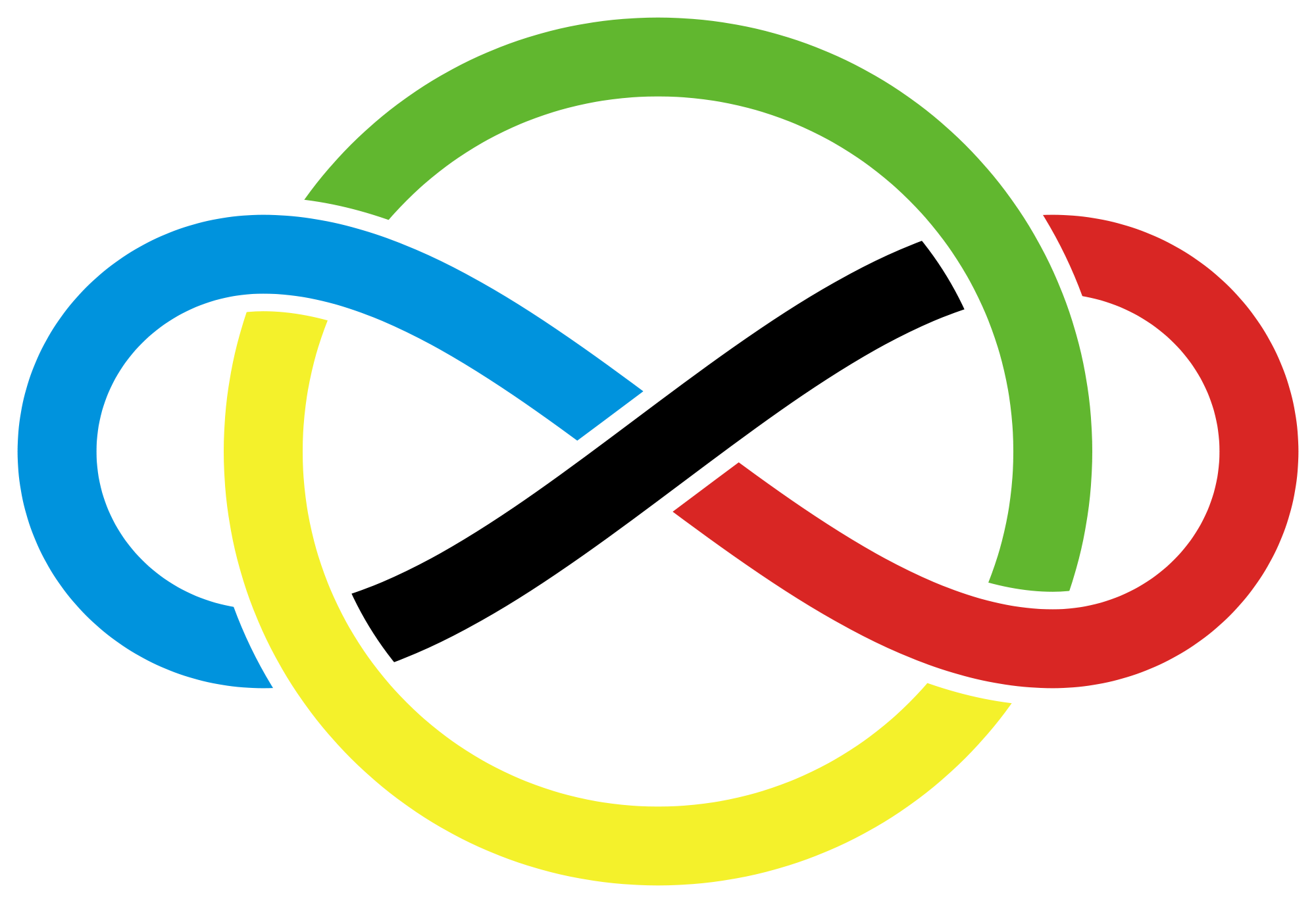# IMO Problem 2

Algebra Level 4For what real values of $x$ is

$\sqrt{x+\sqrt{2x-1}} + \sqrt{x-\sqrt{2x-1}} = A$,

given $A=2$, where only non-negative real numbers are admitted for square roots?

This problem is from the IMO.This problem is part of this set.

×

Problem Loading...

Note Loading...

Set Loading...